# Cm to inches

1 cm = 0.3937 inch and 1 inch = 2.54 cm. Use a simple formula, the CONVERT function or download our free unit converter to convert from cm to inches or vice versa (see below).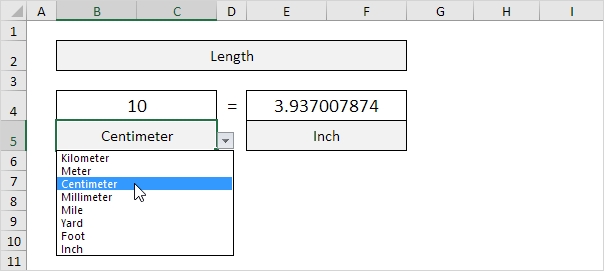1. First, to convert from centimeters to inches, divide by 2.54. The simple formula below does the trick.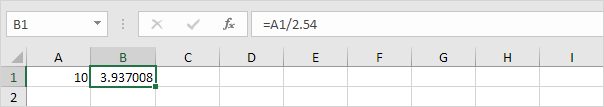2. To convert from inches to centimeters, multiply by 2.54.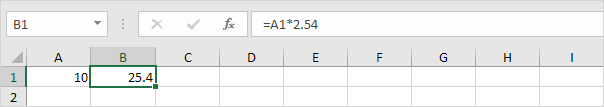3. You can also use the CONVERT function in Excel to convert from centimeters to inches.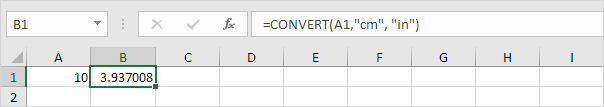Note: the CONVERT function has three arguments (number, from_unit and to_unit).

4. You can also use the CONVERT function in Excel to convert from inches to centimeters.Download our free unit converter to quickly and easily convert from cm to inches (or vice versa).

5. Select Length from the category drop-down list.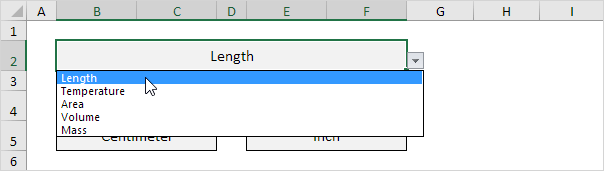Explanation: because you selected Length from this drop-down list, the dependent drop-down lists in cell B5 and cell E5 now contain length units.

6. Enter a number and select the correct length units.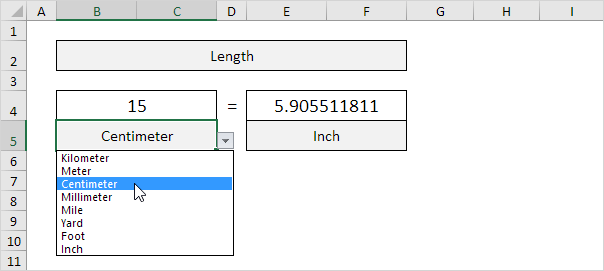Explanation: the unit converter uses worksheet change events to automatically execute VBA code when you change a cell (B2, B4, B5, E4 or E5). The VBA code uses the CONVERT function to convert from one measurement unit to another and uses the VLOOKUP function to lookup the correct unit abbreviations (cm, in, yd, ft, etc.) stored on the second worksheet.

Go to Next Chapter: Keyboard Shortcuts